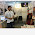### Lecture 3: The conditional and the Biconditionals Statements and Binary System

25.06.12
The conditional and the Biconditionals Statements.

If the connectors$\rightarrow$ is used between any two statements P and Q to form a compound statement P$\rightarrow$ Q (reads if P then Q), the statement is called a conditional statement.
Example:
Let P = You Passed English
Q = You will graduate

Then this can be recorded in Truth Table as:
 P Q P$\rightarrow$Q T T T T F F F T T F F T

The statement P$\rightarrow$Q reads if you pass English then you will graduate. This statement is false only when you pass English (true) but you will not graduate. Therefore the final column will be true in every position but the second.

The connective$\leftrightarrow$ is called the biconditional and may be placed between any two statements to form a compound statement P$\leftrightarrow$ Q (reads P if and only if Q).

Then this can be recorded in Truth Table as:
 P Q P$\leftrightarrow$Q T T T T F F F T F F F T

THE BINARY SYSTEM AND BOOLEAN ALGEBRA
The Boolean algebra provides rigorous procedures for deciding whether a statement is true or false;if the statement can be expressed in two variables. In Boolean algebra true is represented by a 1 and false by a 0. With these two digits (0,1) and the three basic operations called “not”, “and” and “or”, digital algebra or switching algebra was developed.

The basic operations and their meaning:

 Operation Meaning Symbol or Determine a single input bit from the values of two or more input + (A+B) and Determines a single input bit from the value of two or more input . (A.B or AB) not Change binary bits to its opposite value ! (!A or ~A or bar over A)

Any relationship between logical variables are called logical expressions. These expressions can be written as an equation for example the equation A + B + C = F where F is the name of the output variable. The expression A + B + C = F expresses the action of and/or function. Through Boolean Algebra logical analysis can be performed using these three functions.
The electronic representation of these functions are called logic gates. There are the and gate the not and the or gates. These logic gates are basic functional units for both arithmetic and logic operations; to operate they must accept binary numbers, and should have a carry bit of one or 0, (from the adjacent lower power of two), and should produce as outputs a sum bit and a carry bit for the next higher power of two.

1.The table is bit confusing please can anyone help me out to elaborate the table in a simple of the basic operations and their meaning....???

1.There are 3 tables on this page, which one you want to be explained?

2.The basic operations and their meaning:

Operation Meaning Symbol
or Determine a single input bit from the values of two or more input + (A+B)
and Determines a single input bit from the value of two or more input . (A.B or AB)
not Change binary bits to its opposite value ! (!A or ~A or bar over A)

this one...

1.This table shows working of basic operations and their meanings. These meanings can be read as A + B, this is AND which requires both inputs to generate output, A.B, this is OR which requires either input to generate output, and !A is not A or inverse of A, which is NOT gate.